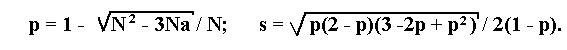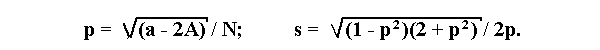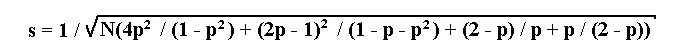## Equations for estimation of recombination fraction between a marker and a sporophytic lethal in F2 progenies with the maximum likelihood method

 Kosterin, O.E., Berdnikov, V.A. and Gorel, F.L. Institute of Cytology and Genetics Novosibirsk 630090 Russia

If a lethal is linked to a recessive allele a of a marker, then the maximum likelihood estimation p of the recombination fraction and its standard deviation s will be determined by the following formulae:where N is the total number of progeny, a is the number of individuals with recessive phenotype.
If a lethal is linked to a dominant allele A, then the formulae become:where N is the total number of progeny, A is the number of individuals with dominant phenotype and a is the number of individuals with recessive phenotype.
If a lethal is linked to an allele B of a co-dominant marker with alleles A and B then p is to be found by a numerical solution of the following equation:

2 Np5- (6A +5H +4B)p4+ 2(3A + B)p3+ (5H - 4A + 2B)p2- 2(H + 2B)p + 2B = 0

where N is the total number of progeny, A, B, and H are the numbers of genotypes A/A, B/B, and A/B, respectively.
The standard deviation s is: